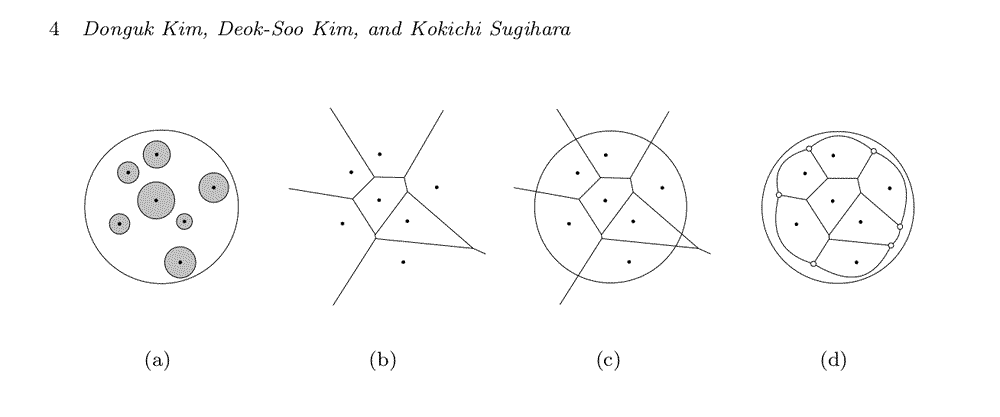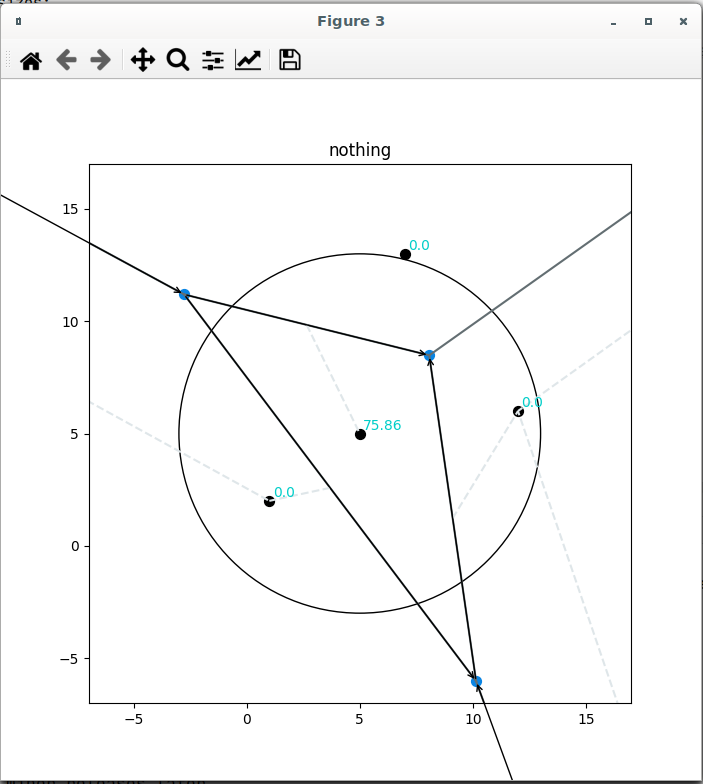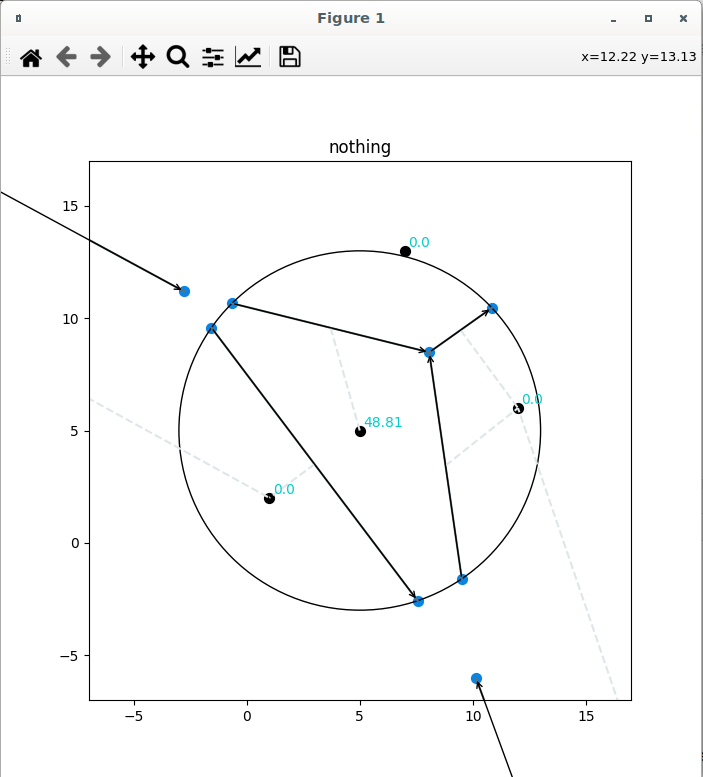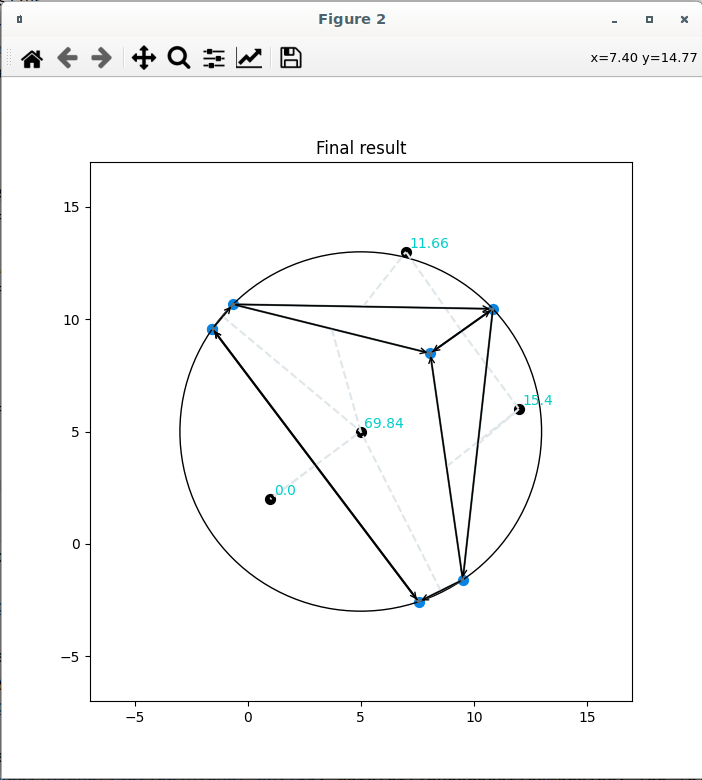# Disc packing problem: Circular bounding box for voronoi diagrams

Still working on the disc packing problem. I need a seed for the Voronoi disc algorithm first.

In the last blog entry I motivated why I am building a Python solution for closest disc packing.

### The challenge:

The Voronoi algorithm for discs  is based on the Voronoi algorithm for points.To generate a voronoi diagram for discs you have to go three steps.

1) Take the midpoints of your discs

2) Make a voronoi diagram of the midpoiints

3) Clip your voronoi diagram on a circle to gain a seed for the disc algorithm.

## The Theory

So my first challenge was to generate the vertices of figure d from the Voronoi diagram of figure c.

You may have noticed that our diagram (d) does not look coorect. The autors of  claim that only the topology of the edges connecting the clipped edges is important: So I shortcut to a simple circular clippling without modelling the real shape of the outlying edges.

### Our Implementation

The implementation of Fortune's algorithm done by Yatoom  was the most promising candidate since hir already implemented a bounding polygon in hir code.

I extended Yatooms code by an implementation for a circular bounding box . This implementation is quite cumbersome code ATM since I struggled with my math for some days.

Also my code is not well documented since this is preliminary code to play with. I added some visualization code to show the steps of finding the circular bounding points. So please set DEBUG=True in the bounding_circle.py if you are lost.

This is the Voronoi diagram we start with.  We have four iniitial points (black dots). The Yatoom implemtation of Fortunes Algorithms gives us the Voronoi diagram (Blue dots and vectors for the edges).Our clipping algorithm at work. Please note the most nasty edge (left, down) which has two points ouside the circle and is clipped correctly.It took me some days to figure out the orientation of the half-Edges Yatoom based its code on. I will have a deep thinking about this all before we can go to optimization.The final result. The Voronoi diagram is clipped correctly at the circular boundary.

As you may have noticed the dotted lines are not consistent pointing toward the edges of the cell. These artefacts I noticed before extending Yatooms code. So these may be errors based of the original code.

 https://www.researchgate.net/publication/220669836_Euclidean_voronoi_diagram_for_circles_in_a_circle

 https://github.com/Yatoom/voronoi

 https://github.com/Inqbus/voronoi